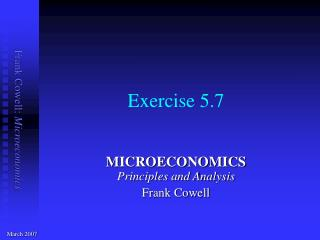Download PresentationExercise 5.7

# Exercise 5.7 - PowerPoint PPT PresentationDownload Presentation## Exercise 5.7

- - - - - - - - - - - - - - - - - - - - - - - - - - - E N D - - - - - - - - - - - - - - - - - - - - - - - - - - -
##### Presentation Transcript

1. Exercise 5.7 MICROECONOMICS Principles and Analysis Frank Cowell March 2007

2. Ex 5.7: Question • purpose: Illustration of labour supply model with very simple preferences • method: Consumer optimisation with endogenous budget constraint

3. Ex 5.7: Worker’s problem • Basic constraints on worker are • x is consumption • `y is non-labour income • w is wage rate • ℓ is labour supply • Worker’s problem can therefore be written as • found by substituting from above into utility function

4. Ex 5.7: Worker’s optimum • Take log of maximand to get • a log(wℓ +`y) + [1  a] log(1  ℓ) • Differentiate with respect to ℓ • This is zero if • wℓ + aw + [a 1]`y = 0 • which implies ℓ = a + [a 1]`y / w • But this only makes sense if ℓ is non-negative • requires w≥ [1 a]`y / a • so optimal labour supply is

5. slope = w Ex 5.7: Interior maximum • (leisure, consumption)-space x • Max value of leisure • Indifference curves • Non-labour income • Budget constraint • Optimum • w≥ [1 a]`y / a • 0 < ℓ*< 1 `y 1  ℓ 0 1  ℓ* 1

6. Ex 5.7: Corner solution • Indifference curves as before x • Non-labour income • Budget constraint • Optimum • w< [1 a]`y / a • ℓ*= 0 • `y 1  ℓ 0 1

7. Ex 5.7: Points to note • Treat leisure as a conventional good • Has a natural upper bound • where labour is zero • Have to allow for corner solution • where person chooses not to work • Type of equilibrium depends on • wage rate • non-labour income